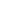| lesson material | material summary | questions and answers | definitions | types and examples | other information | materi pelajaran | ringkasan materi | pertanyaan dan jawaban | definisi | jenis-jenis dan contoh-contoh | informasi lainnya |

# Draw Objects with Loops and Random Functions in HTML5 Canvas

In the article introducing HTML5 Canvas before, we have learned how to understand basic objects such as points, lines, squares, circles, and triangles.
Obejek is drawn only once.
How about if we want to draw lots of objects and draw them randomly?
To answer this question, we must use repetition and function `random()`.
For more details, let's discuss ...

## Draw Objects with Repetition

In the example in the previous article, we have used the square twice.
``````<script type="text/javascript">
var canvas = document.getElementById("myCanvas");
var ctx = canvas.getContext("2d");

ctx.strokeRect(10,10,100,100);
ctx.fillRect(120,10,100,100);
</script>
``````
With results like this: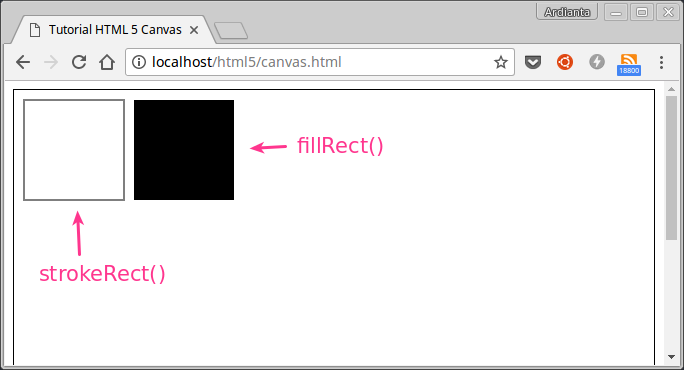Then what if we want to draw 10 square?
We can just retype the function to draw it as much `10x`.
``````ctx.strokeRect(10,10,100,100);
ctx.strokeRect(110,10,100,100);
ctx.strokeRect(210,10,100,100);
ctx.strokeRect(310,10,100,100);
ctx.strokeRect(410,10,100,100);
ctx.strokeRect(510,10,100,100);
ctx.strokeRect(610,10,100,100);
ctx.strokeRect(710,10,100,100);
ctx.strokeRect(810,10,100,100);
ctx.strokeRect(910,10,100,100);
``````
Then if you want as many pictures `1000x`?
Is it able to type as much code as possible `1000`?
It must be tired of typing 1000 lines with different values `x`and `y`ones.
therefore, we must use repetition.
Example :
We will describe 5 squares with `x`increasing values `10`for each loop and `y`remain.
``````<script type="text/javascript">
var canvas = document.getElementById("myCanvas");
var ctx = canvas.getContext("2d");

for(x = 10; x <= 50; x+=10){
ctx.strokeRect(x, 10, 10, 10);
}
</script>
``````
We will bar square with size `10x10`at point `y=10`and `x`change.
Each loop of the x value will increase `10`.
``````perulangan ke 1, nilai x = 10 dan y = 10
perulangan ke 2, nilai x = 20 dan y = 10
perulangan ke 3, nilai x = 30 dan y = 10
perulangan ke 4, nilai x = 40 dan y = 10
perulangan ke 5, nilai x = 50 dan y = 10
``````
If we state it in mathematical straight-line equations:
``````y = 10 (nilai y tetap 10)
``````
* recall math lessons (straight line equations, functions, and linear algebra)The result: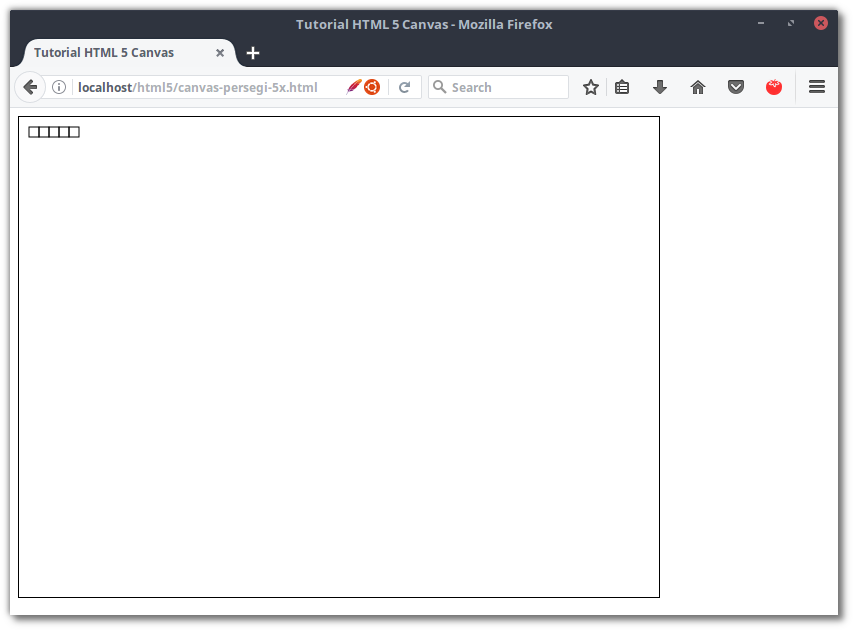Now try as many pictures as `50x`:
``````<script type="text/javascript">
var canvas = document.getElementById("myCanvas");
var ctx = canvas.getContext("2d");

for(x = 10; x <= 500; x+=10){
ctx.strokeRect(x, 10, 10, 10);
}
</script>
``````
The result: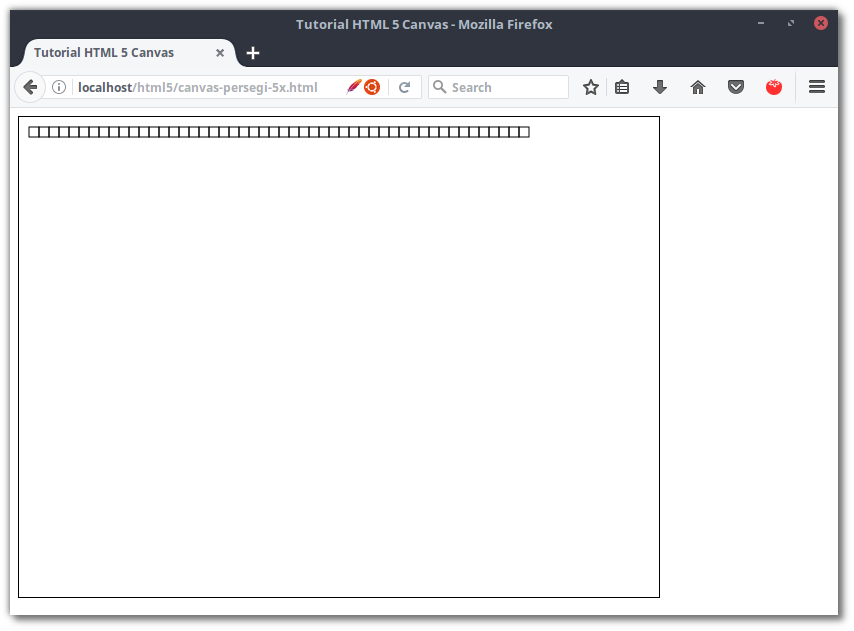Then what if the `y`current values ​​change.
It's easy, we just change it to like this:
``````<script type="text/javascript">
var canvas = document.getElementById("myCanvas");
var ctx = canvas.getContext("2d");

for(y = 10; y <= 500; y+=10){
ctx.strokeRect(10, y, 10, 10);
}
</script>
``````
Then the result: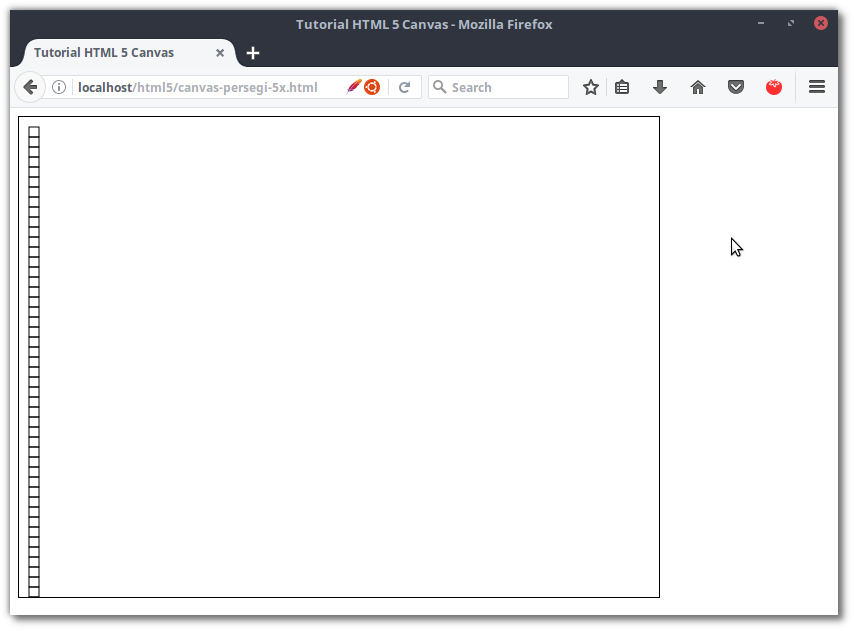Still want more?
Let's try both changes (value `x`and `y`change).
``````<script type="text/javascript">
var canvas = document.getElementById("myCanvas");
var ctx = canvas.getContext("2d");

for(i = 10; i <= 500; i+=10){
ctx.strokeRect(i, i, 10, 10);
}
</script>
``````
Value `x`and `y`we take from variables `i`.
The result: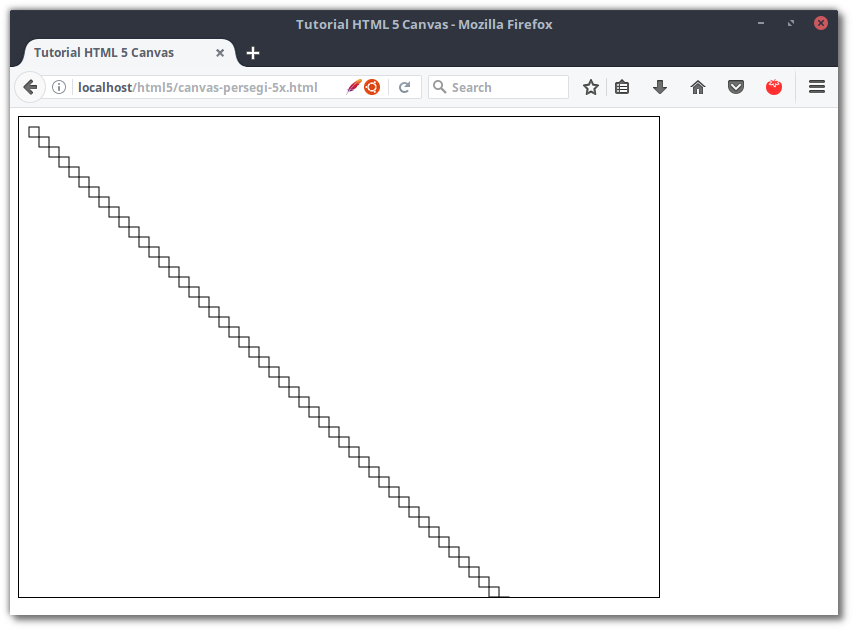Line drawings as above can be expressed with mathematical equations:
``````y = x
``````

### Using Nested Repetition

Nested loop means repetition in repetition.
Now let's try to draw as many squares `40`in the direction `x`(horizontal) and `40`ka direction `y`(vertical) with nesting loops.
``````<script type="text/javascript">
var canvas = document.getElementById("myCanvas");
var ctx = canvas.getContext("2d");

for(x = 10; x <= 400; x+=10){
for(y = 10; y <= 400; y+=10){
ctx.strokeRect(x, y, 10, 10);
}
}
</script>
``````
So many of the squares that will be drawn are `1600`square fruit.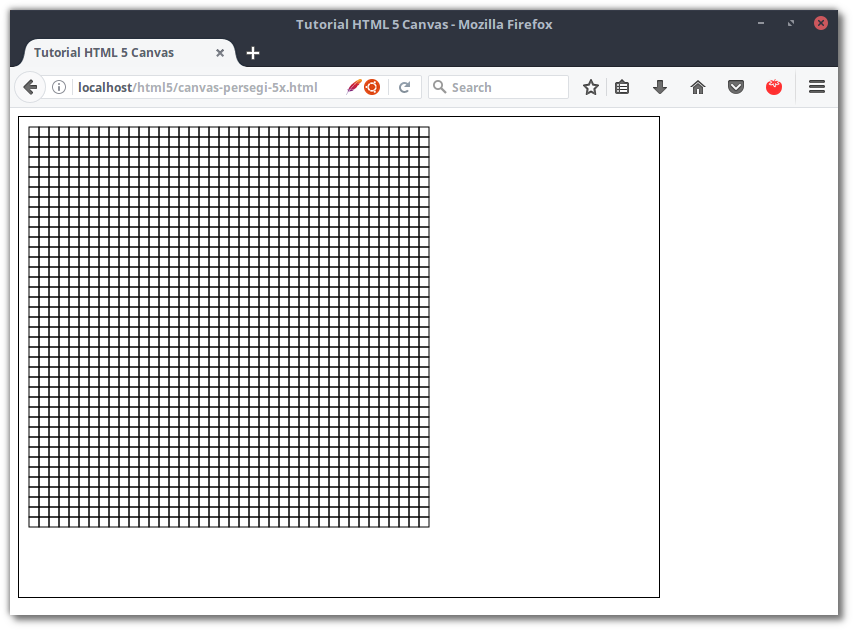Now what if the value `y`on our 2nd loop sets the initial value is `x`.
``````<script type="text/javascript">
var canvas = document.getElementById("myCanvas");
var ctx = canvas.getContext("2d");

for(x = 10; x <= 400; x+=10){
for(y = x; y <= 400; y+=10){
ctx.strokeRect(x, y, 10, 10);
}
}
</script>
``````
The result: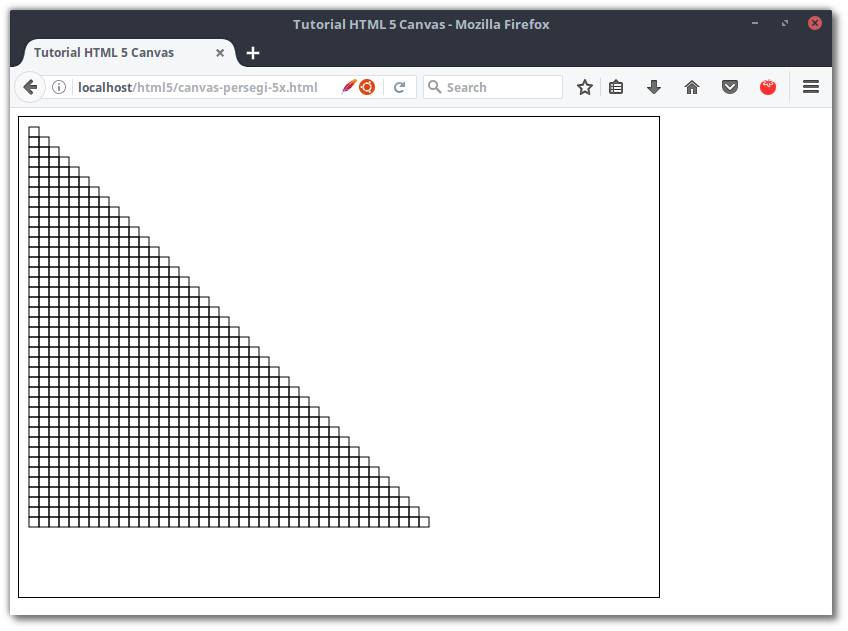Next I will show the results of my creation that you can follow and please think about how the image can be formed.
Code:
``````<script type="text/javascript">
var canvas = document.getElementById("myCanvas");
var ctx = canvas.getContext("2d");

for(x = 10; x <= 400; x+=10){
for(y = 10; y <= 400; y+=10){

if((y*x) % 200 != 0){
ctx.strokeRect(x, y, 10, 10);
} else {
ctx.fillRect(x, y, 10, 10);
}

}
}
</script>
``````
* operator `%`functions to calculate the remainder for.
The result: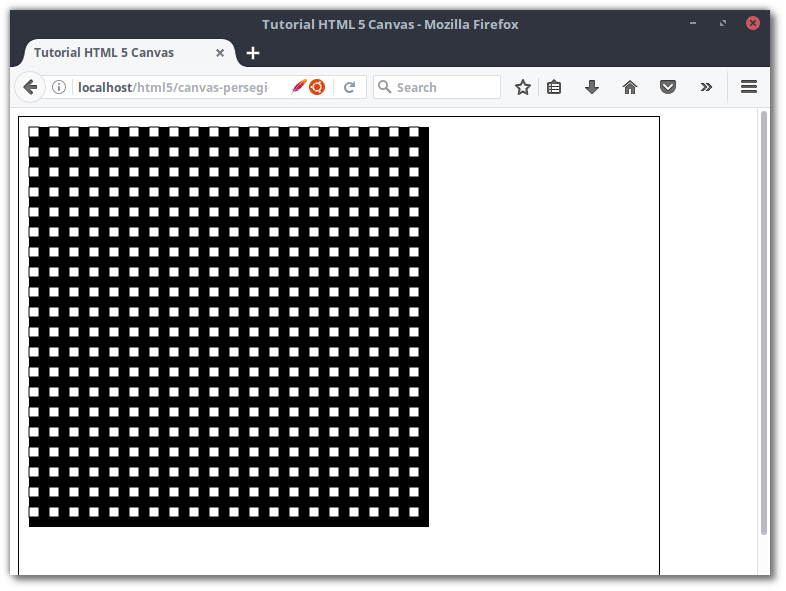Code:
``````<script type="text/javascript">
var canvas = document.getElementById("myCanvas");
var ctx = canvas.getContext("2d");

for(x = 10; x <= 400; x+=10){
for(y = 10; y <= 400; y+=10){

if((y*x) % 21 == 0){
ctx.strokeRect(x, y, 10, 10);
} else {
ctx.fillRect(x, y, 10, 10);
}
}
}
</script>
``````
Results: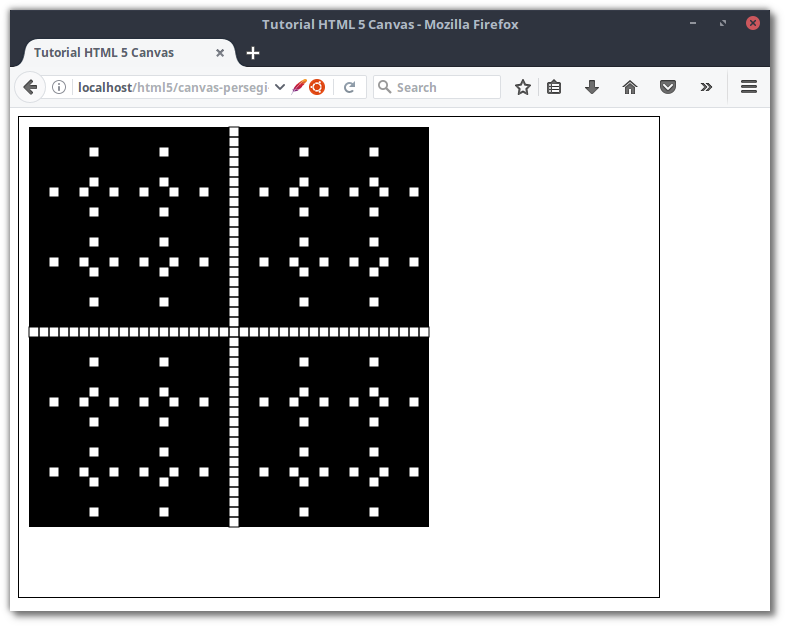That's all ... so that this tutorial is not extended.

## Drawing Objects with Math.random () Functions

Function `random()`is a function that produces random values ​​from `0,0`up to `1,0`We will use this function to determine the value `x`and `y`so that the object we are drawing is randomly placed on the canvas .
Here is an example of the value generated by the function `random()`.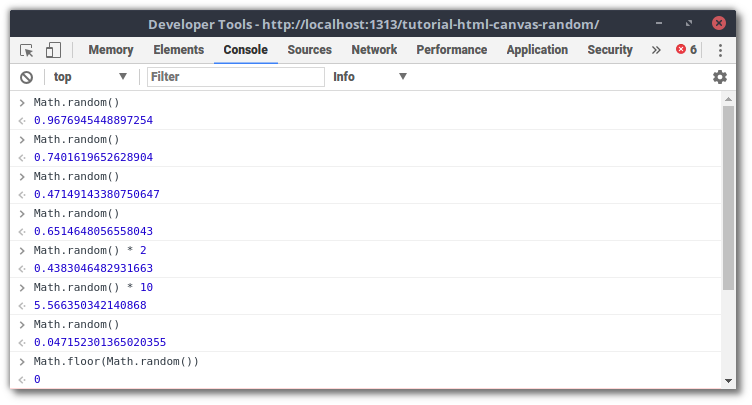Because the function `random()`produces a value `0,xxx`, we have to convert it to a decimal number (integer) .
The way we can use the function is `floor()`to round the value.
For example like this:
``````Math.floor(Math.random() * 5)
``````
Then the random value we will get is from `0`up to `5`.
Now let's try to draw a point on the canvas with a value `x` and `y`random from the `0`length of the canvas.
``````<script type="text/javascript">
var canvas = document.getElementById("myCanvas");
var ctx = canvas.getContext("2d");

for(i = 0; i < canvas.width; i++){
for(j = 0; j < canvas.height; j++){

// isi nilai titik dengan bilangan acak
var x = Math.floor(Math.random() * canvas.width);
var y = Math.floor(Math.random() * canvas.height);

// gambar titknya
ctx.fillRect(x, y, 1,1);

}
}
</script>
``````
The result: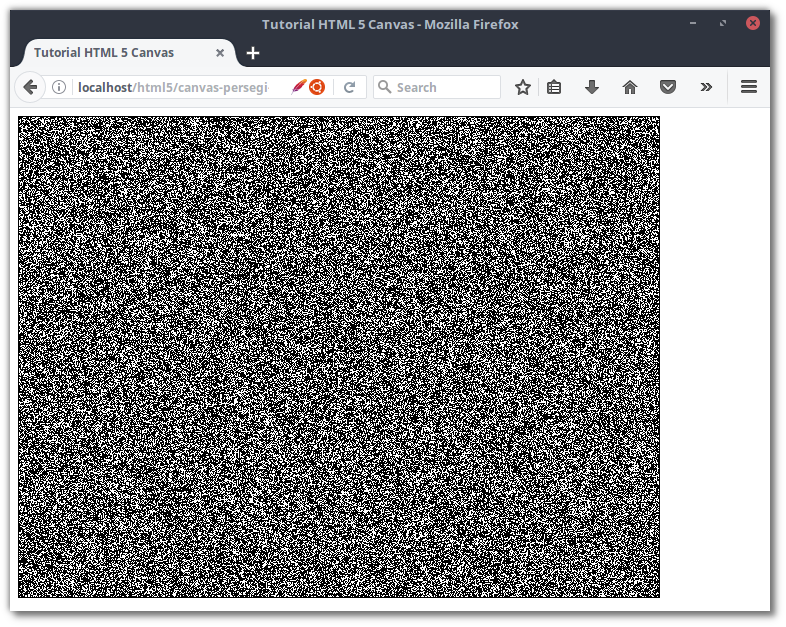Steady! It seems like the point is too much.
Try to draw as many points as `10000`or `100x100`.
``````<script type="text/javascript">
var canvas = document.getElementById("myCanvas");
var ctx = canvas.getContext("2d");

for(i = 0; i < 100; i++){
for(j = 0; j < 100; j++){

// isi nilai titik dengan bilangan acak
var x = Math.floor(Math.random() * canvas.width);
var y = Math.floor(Math.random() * canvas.height);

// gambar titknya
ctx.fillRect(x, y, 1,1);

}
}
</script>
``````
The result: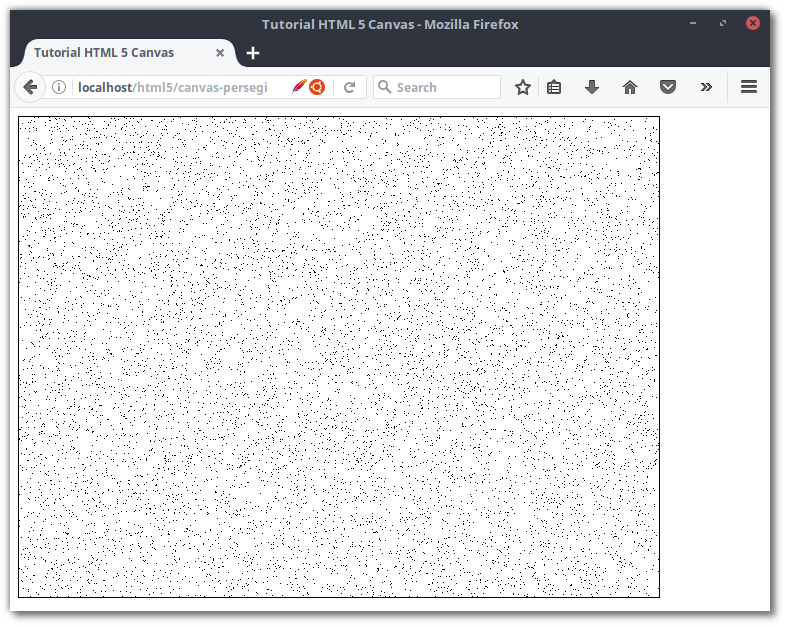Next we modify the code.
Please increase the size of the square to `10x10`and image as many `200`times. We use two squares, which are `strokeRect()`as many `100`and `fillRect()`as many `100`.
``````<script type="text/javascript">
var canvas = document.getElementById("myCanvas");
var ctx = canvas.getContext("2d");

for(i = 0; i < 10; i++){
for(j = 0; j < 10; j++){

// isi nilai titik dengan bilangan acak
var x = Math.floor(Math.random() * canvas.width);
var y = Math.floor(Math.random() * canvas.height);

// gambar perseginya
ctx.fillRect(x, y, 10,10);

}
}

for(i = 0; i < 10; i++){
for(j = 0; j < 10; j++){

// isi nilai titik dengan bilangan acak
var x = Math.floor(Math.random() * canvas.width);
var y = Math.floor(Math.random() * canvas.height);

// gambar perseginya
ctx.strokeRect(x, y, 10,10);

}
}
</script>
``````
The result: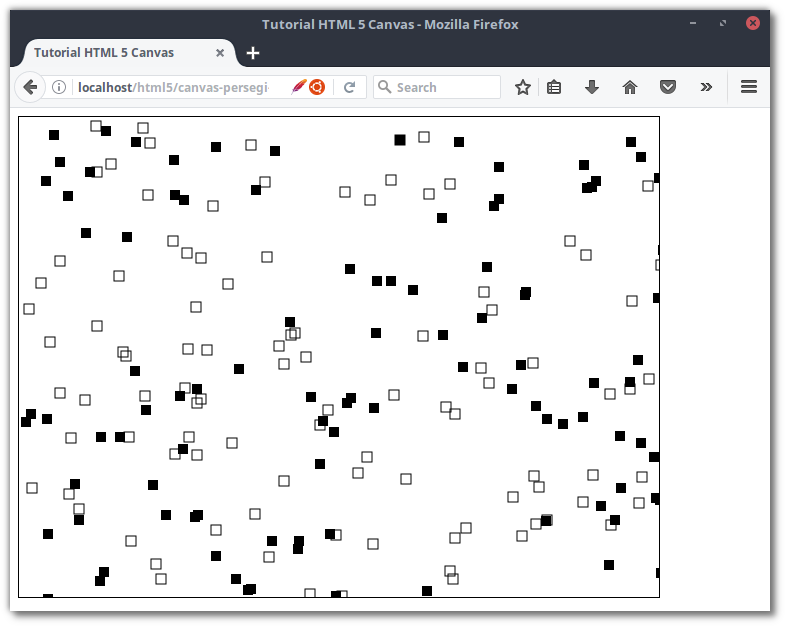Try also to be creative with circles.
``````<script type="text/javascript">
var canvas = document.getElementById("myCanvas");
var ctx = canvas.getContext("2d");

for(i = 0; i < 10; i++){
for(j = 0; j < 10; j++){

// isi nilai titik dengan bilangan acak
var x = Math.floor(Math.random() * canvas.width);
var y = Math.floor(Math.random() * canvas.height);

// gambar lingkaran
ctx.beginPath();
ctx.arc(x, y, 20, 0, 2*Math.PI);
ctx.stroke();

}
}
</script>
``````
The result: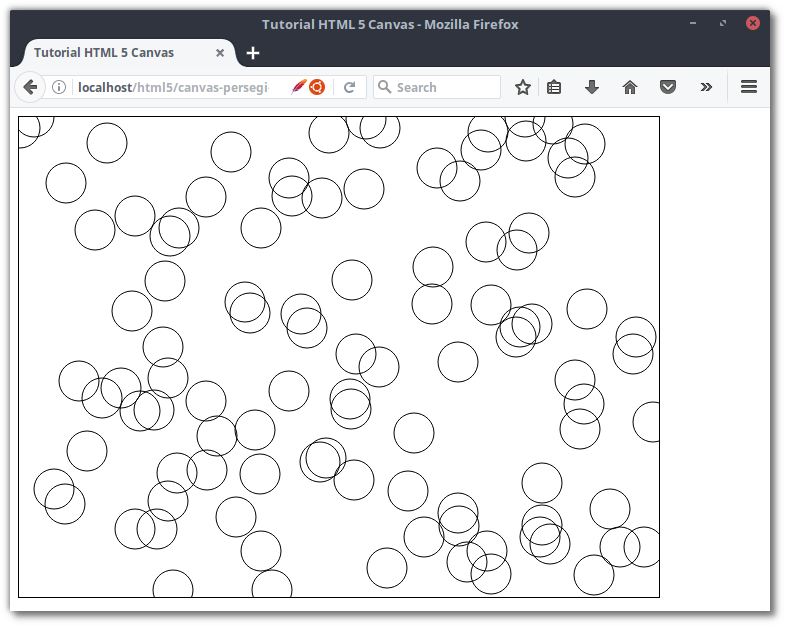Try also the fingers we love random, eg from `5`up to `30`.
``````<script type="text/javascript">
var canvas = document.getElementById("myCanvas");
var ctx = canvas.getContext("2d");

for(i = 0; i < 10; i++){
for(j = 0; j < 10; j++){

// isi nilai titik dengan bilangan acak
var x = Math.floor(Math.random() * canvas.width);
var y = Math.floor(Math.random() * canvas.height);

// jari-jari random dari 5 sampai 30
var r = Math.floor( Math.random() * (30 - 5) + 5 );

// gambar lingkaran
ctx.beginPath();
ctx.arc(x, y, r, 0, 2*Math.PI);
ctx.stroke();

}
}

</script>
``````
The result: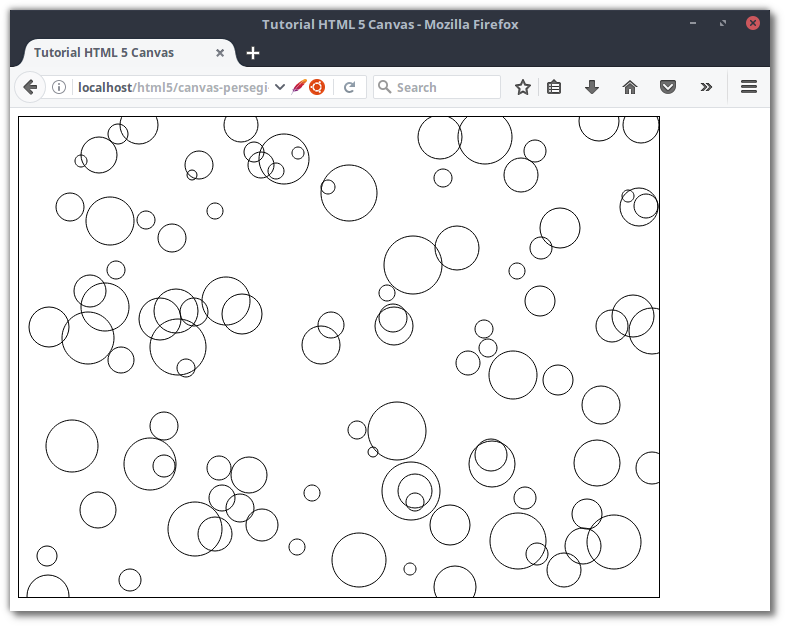## The final word…

That's how to draw objects with repetitions and random functions. Random functions are sometimes used in Games to draw objects in random positions.
For example, the Flappy Bird Game, the barrier pipe is placed randomly with random functions.
On the next occasion we might discuss color and animation.
Thank you for following this tutorial. If there are questions or additions please submit in the comments.
Congratulations and imagine the code.
Reference :
##### 0 Komentar untuk "Draw Objects with Loops and Random Functions in HTML5 Canvas"

Silahkan berkomentar sesuai artikel

Template By Kunci Dunia